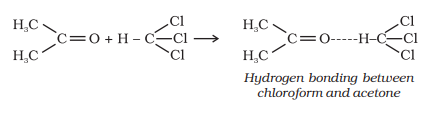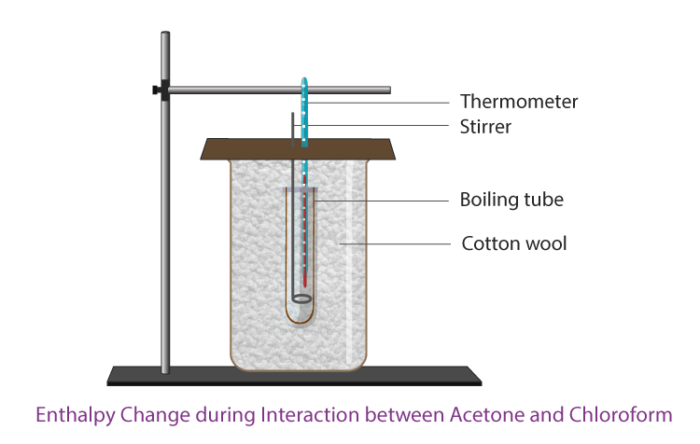# Enthalpy Change during Interaction between Acetone and Chloroform

Heat evolves when acetone is mixed with chloroform, due to the formation of hydrogen bonds between chloroform and acetone. The heat evolved during this interaction can be determined experimentally using a calorimeter to mix the two liquids and measure the heat change.

## Aim:

To determine the enthalpy change during interaction (Hydrogen bond formation) between Acetone and Chloroform.

## Theory:

Liquid pairs display a departure from ideal behavior when mixing. Acetone and chloroform form a non-ideal liquid pairing system, showing negative deviations from the law of Raoult law. Acetone-chloroform mixture is a prominent example of a system with a marked negative deviation from the behaviour of the ideal solution. So it was the subject of numerous theoretical and experimental investigations.

When acetone and chloroform are mixed together heat is evolved due to the formation of hydrogen bonds between chloroform and acetone.

The corresponding chemical equation is given below.This hydrogen bonding lowers the escaping tendency of both molecules and as a result, the vapor pressure of the solution is less than that expected from Raoult’s law. On the other hand, in the pure state, only the weak forces of Van der waal hold both chloroform and acetone molecules.

Change in enthalpy occurs in this process due to the formation of hydrogen bonds. The change in the heat for the specified amount is reported. Therefore enthalpy changes are reported when 1 mol chloroform is mixed with 1 mol acetone.

## Materials Required:

1. Measuring cylinder
2. Thermometer
3. Glass rod
4. Cotton wool
5. Piece of cardboard
6. Stirrer
7. Boiling tube
8. Beaker
9. Chloroform
10. Acetone

## Apparatus Setup:## Procedure:

1. Calculate the water equivalent of the calorimeter or beaker.
2. Take 50ml of chloroform in a beaker and 50ml of acetone in another beaker.
3. Note down the initial temperature of both the solutions.
4. Transfer the chloroform volume equivalent to 0.1 mol (almost 8.14 mL) to the insulated boiling tube after measuring from a measuring cylinder,
5. Transfer the volume of acetone in a clean measuring cylinder equivalent to 0.1 mol of acetone (almost 7.34 mL).
6. Pour in the chloroform contained in the isolated boiling tube acetone from the measuring cylinder.
7. Stir the mixture rapidly and note down the final temperature.
8. Calculate the rise in temperature.

## Observation and Inference:

 Initial temperature of acetone and chloroform t1oC Final temperature of acetone and chloroform t2oC Change in temperature (t1-t2)oC Water equivalent of calorimeter W g Density of acetone 0.787 g/cm3 Density of chloroform 1.499 g/cm3 Specific heat of acetone S2 2.18 J/g Specific heat of chloroform S1 0.96 J/g

## Calculations:

Enthalpy change can be calculated as below.

= $$W\times 4.2\times (t_{1}-t_{2}) + [100\times 1.499\times S1+100 \times 0.787\times S_{2}] (t_{1}-t_{2})$$Joules

= _______ Joules.

## Results and Discussions:

The enthalpy change during the mixing of 100ml of acetone with 100ml of chloroform is _________ Joules.

## Precautions:

1. For thermal insulation, cotton should be used.
2. The mixture of acetone and chloroform should be stirred carefully.
3. Measure carefully chloroform and acetone.
4. Record very carefully the temperature with a graduated thermometer up to 0.1°C.

## Frequently Asked Questions on Enthalpy Change during Interaction between Acetone and Chloroform

### What will be the enthalpy of a system if heat is transferred at constant pressure?

It increases, At constant pressure, (dQ)=dh where h=u+pv is the specific enthalpy of the system.

### Is the enthalpy of an ideal gas depends on its temperature?

Yes enthalpy depends on the temperature of the system because the internal energy of an ideal gas depends only on the temperature.

### Which device is used to determine the dissolution enthalpy of a particular salt?

The device is used is calorimeter to determine the enthalpy of salt dissolution.

### What is thermochemistry?

Thermochemistry is the study of the associated heat energy with chemical reactions and/or physical transformations. A reaction can release or absorb energy, and like melting and boiling, a phase change can do the same.

### Define enthalpy of solution.

Enthalpy of a solution or heat of solution is the amount involved in dissolving a mole or sometimes a gram in a large excess of solvent

Take up a quiz on Enthalpy Change during Interaction between Acetone and Chloroform